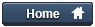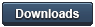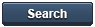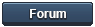### Gammon Forum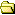Entire forum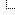Electronics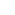Microprocessors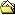Function pointers / function callbacks / function variables

Function pointers / function callbacks / function variables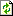Refresh page

Posted by Nick Gammon   Australia  (22,921 posts)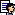bio   Forum Administrator
Date Tue 23 Sep 2014 11:50 PM (UTC)

Amended on Sat 04 Jun 2016 04:18 AM (UTC) by Nick Gammon

Message

Introduction

This post addresses the question that appears quite often on the Arduino forum:

Quote:

How do I call different functions depending on a decision at runtime?

Or:

Quote:

How can I make a variable that holds a function?

The general idea is something like this:

``````
int action = 3;   // 3 is an example

if (action == 0)
doAction0 ();
else if (action == 1)
doAction1 ();
else if (action == 2)
doAction2 ();
else if (action == 3)
doAction3 ();
else if (action == 4)
doAction4 ();
``````

These actions might be to display a different LED pattern, play a different tune, etc.

Now of course you can use switch / case like this:

``````
int action = 3;   // 3 is an example

switch (action)
{
case 0: doAction0 (); break;
case 1: doAction1 (); break;
case 2: doAction2 (); break;
case 3: doAction3 (); break;
case 4: doAction4 (); break;
} // end of switch
``````

But even that can get tedious after a while.

The poster usually wants to do something like this:

``````
// THIS WON'T WORK!
action = 3;
doAction'action' ();  // call doAction3 ();
``````

Or:

``````
// THIS WON'T WORK!
action = 3;
doAction + action (); // call doAction3 ();
``````

Where somehow the action number gets magically attached to the function name. However this doesn't work (nor does it compile even) because at runtime the code does not know the names of the functions.

Function pointers

This is where function pointers come in handy. They are variables that point to functions.

First, it is very helpful to make a typedef which declares the sort of function you are planning to call. For example:

``````
typedef void (*GeneralFunction) ();
``````

In this case we are planning to call a function which takes no arguments, and returns void. The following function declaration would match that type:

``````
void doAction1 ()
{
Serial.println (1);
}
``````

Make an instance of the function pointer

Now that we have a type which can point to a function, we can make an instance of that type (in other words, a variable that points to a function):

``````
GeneralFunction foo;
``````

Tip:
The variable name foo is just an example name. Feel free to choose a suitable name for your application. See Foo (Wikipedia) for more details about the use of "foo" in tutorials.

Below are some functions that could be assigned to that pointer, as they are declared as having no arguments and no return value:

``````
void hello ()
{
Serial.print ("hello");
}  // end of hello

void world ()
{
Serial.print ("world");
}  // end of world
``````

Now we are ready to assign a function to the pointer (so the pointer "points" to that function), for example:

``````
foo = hello;
``````

Finally we call the pointed-to function:

``````
foo ();  // prints "hello"
``````

Notice the brackets! You need them to tell the compiler to call the function that foo points to.

We can change what foo points to:

``````
foo = world;
foo ();  // prints "world"
``````

The completed sketch, if you want to try it:

``````
typedef void (*GeneralFunction) ();

void hello ()
{
Serial.print ("hello");
}  // end of hello

void world ()
{
Serial.print ("world");
}  // end of world

GeneralFunction foo;

void setup ()
{
Serial.begin (115200);
Serial.println ();

foo = hello;
foo ();

foo = world;
foo ();
}  // end of setup

void loop () { }
``````

Calling functions that take arguments or have a return value

If the function we are planning to call takes arguments, or has a return value, this must be part of the typedef, for example:

``````
typedef int (*GeneralArithmeticFunction) (const int arg1, const int arg2);
``````

The above example declares a type (GeneralArithmeticFunction) which is a pointer to a function which takes two int arguments, and returns int.

A complete example:

``````
// Generic arithmetic funtion
typedef int (*GeneralArithmeticFunction) (const int arg1, const int arg2);

int Add (const int arg1, const int arg2)
{
return arg1 + arg2;

int Subtract (const int arg1, const int arg2)
{
return arg1 - arg2;
} // end of Subtract

int Divide (const int arg1, const int arg2)
{
return arg1 / arg2;
} // end of Divide

int Multiply (const int arg1, const int arg2)
{
return arg1 * arg2;
} // end of Multiply

void setup ()
{
// make pointers to functions, put them in local variables
GeneralArithmeticFunction fSubtract = Subtract;
GeneralArithmeticFunction fDivide = Divide;
GeneralArithmeticFunction fMultiply = Multiply;

Serial.begin (115200);
Serial.println ();

//  use the function pointers
Serial.println (fSubtract (40, 2));
Serial.println (fDivide (40, 2));
Serial.println (fMultiply (40, 2));
}  // end of setup

void loop () {}
``````

Making a callback function

Now that functions can be pointed to, you can use them as callbacks.

Typically your "main" code will call a library (or other) function, passing to that function a pointer to a "callback" function which is to be called at an appropriate time.

This example illustrates that:

``````
typedef void (*GeneralMessageFunction) ();

void sayHello ()
{
Serial.println ("Hello!");
}  // end of sayHello

void sayGoodbye ()
{
Serial.println ("Goodbye!");
}  // end of sayGoodbye

void checkPin (const int pin, GeneralMessageFunction response); // prototype

void checkPin (const int pin, GeneralMessageFunction response)
{
{
response ();  // call the callback function
delay (500);  // debounce
}
}  // end of checkPin

void setup ()
{
Serial.begin (115200);
Serial.println ();
pinMode (8, INPUT_PULLUP);
pinMode (9, INPUT_PULLUP);
}  // end of setup

void loop ()
{
checkPin (8, sayHello);
checkPin (9, sayGoodbye);
}  // end of loop
``````

The lines in bold are the important ones. First we declare a callback function type (GeneralMessageFunction).

Then we make a couple of instances of such a function (which has no arguments and returns nothing).

Then the checkPin function takes as arguments a pin number, and a function to call, if that pin goes low. This is what you call a callback function. That is to say, the function checkPin "calls back" another function which was supplied to it as an argument.

The extra line (marked "prototype") was necessary because of the way that the Arduino IDE pre-processes your code. Note the semicolon at the end of the prototype line. By supplying a prototype, the Arduino IDE does not attempt to generate one of its own, which it does incorrectly in the case of functions with callback arguments.

Then in loop() we call checkPin first with one callback function (sayHello) and then another callback function (sayGoodbye). That way we can write a single pin-checking function which takes different actions depending on how it is called.

Tip:
The demonstration example above uses the delay function call to keep the code simple, and avoid sending a lot of output to the serial port if the switch is closed. Generally speaking, delay should be avoided in more complex applications, as it blocks other work.

Other examples of callback functions

The Arduino libraries use callbacks in quite a few places. For example, when doing an attachInterrupt:

``````
``````

In this example the function blink is called when external interrupt 0 fires because the relevant pin changes state.

The blink function might be coded like this:

``````
volatile unsigned long stateChangeCount = 0;
{
stateChangeCount++;
}
``````

Here we are supplying to the function attachInterrupt a pointer to another function (blink) which we want called at the appropriate time.

Using a callback function in qsort

The qsort (QuickSort) function lets you sort arbitrary arrays of data. To handle any data type you supply to the qsort function a "compare" callback function which does the correct comparison (eg. integer comparison, string comparison, floating-point comparison).

The callback function is passed a pointer to two elements which it needs to be compared, to find if the first argument is less than, equal to, or greater than, the second argument.

It returns -1 for less than, +1 for greater than, and zero for equal. Example code:

``````
const int COUNT = 10;

int someNumbers [COUNT] = { 7342, 54, 21, 42, 18, -5, 30, 998, 999, 3  };

// callback function for doing comparisons
template<typename T> int myCompareFunction (const void * arg1, const void * arg2)
{
T * a = (T *) arg1;  // cast to pointers to T
T * b = (T *) arg2;

// a less than b?
if (*a < *b)
return -1;

// a greater than b?
if (*a > *b)
return 1;

// must be equal
return 0;
}  // end of myCompareFunction

void setup ()
{
Serial.begin (115200);
Serial.println ();
// sort using custom compare function
qsort (someNumbers, COUNT, sizeof (int), myCompareFunction<int>);
for (int i = 0; i < COUNT; i++)
}  // end of setup

void loop () { }
``````

Output:

``````
-5
3
18
21
30
42
54
998
999
7342
``````

This example also demonstrates using a "template" to make the comparison function (myCompareFunction) work with any type that supports direct comparisons.

So you could, for example, compare an array of floats by changing the sort line to:

``````
qsort (someNumbers, COUNT, sizeof (float), myCompareFunction<float>);
``````

Putting functions into an array

Now that we have got this far, we can see how we might put function pointers into an array. Then we can simply index into the array to find which pointer we want, and then call that.

``````
void doAction0 ()
{
Serial.println (0);
}

void doAction1 ()
{
Serial.println (1);
}

void doAction2 ()
{
Serial.println (2);
}

void doAction3 ()
{
Serial.println (3);
}

void doAction4 ()
{
Serial.println (4);
}

typedef void (*GeneralFunction) ();

// array of function pointers
GeneralFunction doActionsArray [] =
{
doAction0,
doAction1,
doAction2,
doAction3,
doAction4,
};

void setup ()
{
Serial.begin (115200);
Serial.println ();

int action = 3;   // 3 is an example

doActionsArray [action] ();
}  // end of setup

void loop () { }
``````

We now have an array of function pointers (doActionsArray), into which we can index. So if we want a pointer to the function for action 3 we use:

``````
doActionsArray 
``````

However to call that function the extra brackets are needed. That is:

``````
doActionsArray  ();
``````

How to put function pointers into program memory

If you have a lot of function pointers in an array, you can save RAM by putting that array into program memory, like this:

``````
void doAction0 ()
{
Serial.println (0);
}

void doAction1 ()
{
Serial.println (1);
}

void doAction2 ()
{
Serial.println (2);
}

typedef void (*GeneralFunction) ();

// array of function pointers
const GeneralFunction doActionsArray [] PROGMEM =
{
doAction0,
doAction1,
doAction2,
};

void setup ()
{
Serial.begin (115200);
Serial.println ();

int action = 2;   // 2 is an example

// get function address from program memory, call the function

}  // end of setup

void loop () { }
``````

Modified lines in bold. In this case we mark the array of functions as PROGMEM, and then use pgm_read_word to get that address from program memory (rather than RAM), cast it to GeneralFunction type, and then call it.

Tip:
Using PROGMEM for constants (things which don't change) will save on RAM because they don't have to be copied from PROGMEM (where they are held anyway when the Arduino is powered off) into RAM when the program starts. However if you only have a few such constants, the extra code needed to do this probably is not worth the effort.

Lambda functions

Recent versions of the IDE support the C++11 syntax for "lambda" (unnamed) functions, so the example above can be rewritten more compactly:

``````
// array of function pointers
void (*doActionsArray []) () =
{
[] { Serial.println (0); } ,
[] { Serial.println (1); } ,
[] { Serial.println (2); } ,
[] { Serial.println (3); } ,
[] { Serial.println (4); } ,
};

void setup ()
{
Serial.begin (115200);
Serial.println ();

int action = 3;   // 3 is an example

doActionsArray [action] ();
}  // end of setup

void loop () { }
``````

The functions are now declared "inline" in the array, rather than having to invent names for them (like doAction0, doAction1 etc.).

For more about lambda functions see (amongst other places) Lambda Functions in C++11 - the Definitive Guide

- Nick Gammon

www.gammon.com.au, www.mushclient.com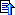top

The dates and times for posts above are shown in Universal Co-ordinated Time (UTC).

To show them in your local time you can join the forum, and then set the 'time correction' field in your profile to the number of hours difference between your location and UTC time.

57,406 views.Refresh page

Go to topic:           Search the forumtop

Quick links: MUSHclient. MUSHclient help. Forum shortcuts. Posting templates. Lua modules. Lua documentation.

Information and images on this site are licensed under the Creative Commons Attribution 3.0 Australia License unless stated otherwise.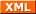Forum RSS feed ( https://gammon.com.au/rss/forum.xml )